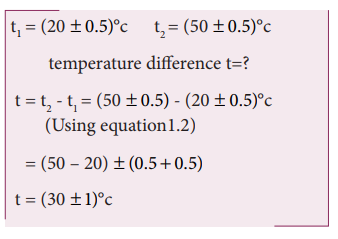Home | | Physics 11th std | Solved Example Problems for Propagation of errors

# Solved Example Problems for Propagation of errors

Physics : Nature of Physical World and Measurement

## (i) Solved Example Problem for Error in the sum of two quantities

Example 1.5

Two resistances R1  = (100 ± 3) Ω, R2  = (150 ± 2) Ω, are connected in series. What is their equivalent resistance?

Solution

Equivalent resistance R = ?

Equivalent resistance R = R1 + R2## (ii) Solved Example Problem for Error in the difference of two quantities

Example 1.6

The temperatures of two bodies measured by a thermometer are t1 = (20 + 0.5)°C, t2 = (50 ± 0.5)°C. Calculate the temperature difference and the error therein.

Solution## (iii) Example Problem for Error in the product of two quantities

Example 1.7

The length and breadth of a rectangle are (5.7 ± 0.1) cm and (3.4 ± 0.2) cm respectively. Calculate the area of the rectangle with error limits.

Solution## (iv) Example Problem for Error in the division or quotient of two quantities

Example 1.8

The voltage across a wire is (100 ± 5)V and the current passing through it is (10±0.2) A. Find the resistance of the wire.

Solution## (v) Example Problem for Error in the power of a quantity

Example 1.9

A physical quantity x is given by xIf the percentage errors of measurement in a, b, c and d are 4%, 2%, 3% and 1% respectively then calculate the percentage error in the calculation of x.

SolutionThe percentage error in x is given byThe percentage error is x = 17.5%

Study Material, Lecturing Notes, Assignment, Reference, Wiki description explanation, brief detail
11th Physics : UNIT 1 : Nature of Physical World and Measurement : Solved Example Problems for Propagation of errors |